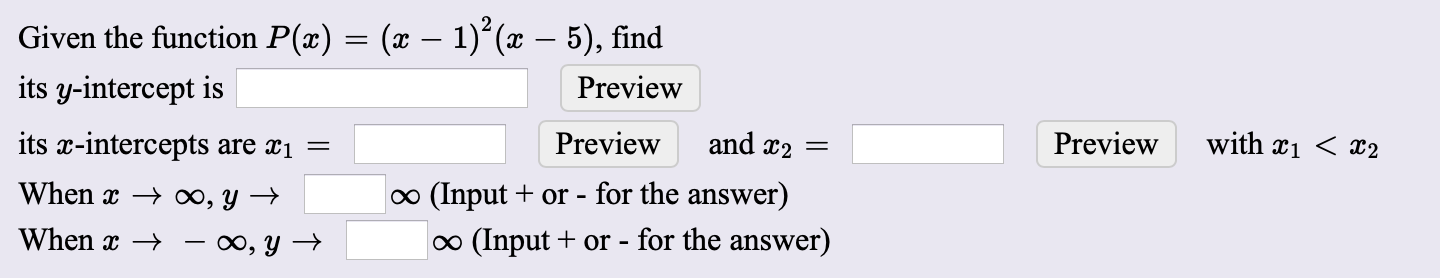# Given the function P(x) = (x – 1)*(x – 5), find its y-intercept is Preview its x-intercepts are x1 Preview and x2 = Preview with xi < x2 When x → ∞, Y → o (Input + or - for the answer) When x → – ∞, y → o (Input + or - for the answer)

Questionhelp_outlineImage TranscriptioncloseGiven the function P(x) = (x – 1)*(x – 5), find its y-intercept is Preview its x-intercepts are x1 Preview and x2 = Preview with xi < x2 When x → ∞, Y → o (Input + or - for the answer) When x → – ∞, y → o (Input + or - for the answer) fullscreen